# Time Dependent Material (Comp. Strength)

Define Compressive Strength of concrete or the change of modulus of elasticity to reflect the variation of modulus of elasticity with time.

The concrete age of each member is measured from the time the concrete is cast, and each member will have an appropriate compressive strength and modulus of elasticity at each construction stage.

If concrete is poured into different parts of a composite section in stages, the difference in compressive strength and modulus of elasticity of each part, due to the difference in concrete age of each part, can be considered.

To reflect the change in compressive strength of concrete or the change in modulus of elasticity, "Variation of Comp. Strength" option should be checked in Construction Stage Analysis Control Data.

From the Main Menu select Properties > Time Dependent > Comp. Strength.

Click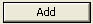to define a new time variant modulus of elasticity (Comp. strength). Click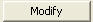and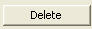to change and delete the data previously entered. Click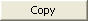to copy previously entered data.

####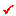Name

Enter the name of function defining the time variant modulus of elasticity (Comp. strength) of concrete.

####Type

Select Code to define the time dependent compressive strength of concrete, or directly specify the numerical values defining the function.

####Development of Strength

When Code is selected in Type, the change of modulus of elasticity is defined by the change of compressive strength.

Code
Select a code defining the change of compressive strength of concrete.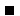Where ACI Code is selectedWhere CEB-FIP(2010) is selectedWhere CEB-FIP(1990) is selectedWhere Ohzagi is selectedWhere INDIA(IRC:18-2000) is selectedWhere European is selectedWhere CEB-FIP(1978) is selected

Specify the Concrete Compressive Strength at 28 Days (fck) and Cement Type (s).Where AS 3600-2009 is selectedWhere AS/RTA 5100.5-2011 is selectedWhere User is selected, enter the modulus of elasticity varying with time under Time and Elasticity in the table.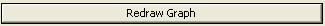: Produce a graph based on the entered data.

Note 1
If User is selected, the entered compressive strength and tensile strength do not affect general analysis, but they are reflected only for the calculation of crack index in heat of hydration analysis.

Note 2
The user may directly input all of modulus of elasticity, compressive strength and tensile strength..

####Scale Factor

Enter a multiplier to apply to the values for modulus of elasticity if User Type is assigned.

####Graph Options

Check on for Log Scale graph.

####Graph Type

The Y-axis of the graph can represent compressive strength, tensile strength or modulus of elasticity.